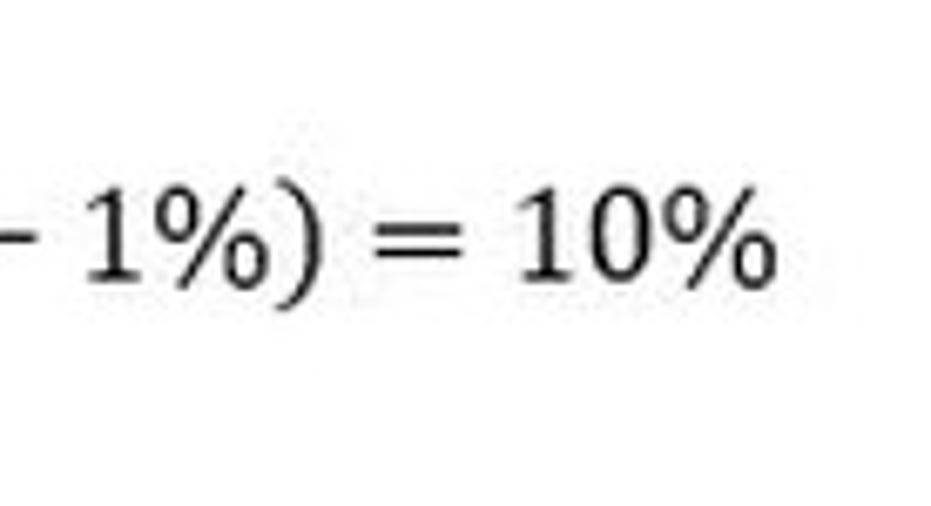How to Calculate Unlevered Cost of CapitalUnlevered cost of capital is the theoretical cost of a company financing itself without any debt.

This number represents the equity returns an investor expects the company to generate, excluding any debt, to justify an investment in the stock. In the real world, however, investors do not explicitly give this number to companies. Instead, the unlevered cost of capital is calculated with a theoretical formula giving an approximation of what the market likely requires.

The formula to calculate unlevered cost of capital

The formula to calculate a company's unlevered cost of capital takes into account the market's risk free rate of return and the risk premium required to invest in that company. The risk free rate is generally tied to the yield on U.S. Treasury bonds. The risk premium is calculated by multiplying the company's unlevered beta by the difference in expected market returns and the risk free rate of return. Beta is a measure of a stock's volatility, and therefore risk, over a period of time. Unlevered beta removes the effects of leverage from the company's beta by adjusting it based on the company's tax rate, debt to equity ratio, and beta.

The formula for unlevered cost of capital is:

Altogether, this formula theorizes that a company will have a higher unlevered cost of capital if investors perceive the stock as being higher risk. Exactly how high, however, will vary based on external market conditions.

An example calculation of unlevered cost of capital

Consider a company with the following capital structure in a market with a risk free rate of 1% and an expected market return of 7%:

Capital Structure Items

Amount

Total Debt

\$100,000

Cost of Debt

5%

Total Equity

\$50,000

Unlevered Beta

1.5

To calculate the company's unlevered cost of capital, we will ignore its debt and the cost of that debt. Calculating the unlevered cost of capital assumes the company has no debt, regardless of whether it does or does not in reality.

Completing the formula from above, the company's unlevered cost of capital is the risk free rate, 1%, plus its 1.5 beta times 7% subtract 1%.

In this example, the company has debt on its balance sheet, and at a cost of just 5% its actual cost of capital will be lower than the 10% unlevered cost of capital calculated. Its unlevered cost of capital is still informative, however. If the company fails to achieve the 10% unlevered returns that investors in this market require, then investor capital is likely to move to alternative investments that do achieve this level. If that happens, supply and demand dictates that the company's stock price would fall as well.

This article is part of The Motley Fool's Knowledge Center, which was created based on the collected wisdom of a fantastic community of investors. We'd love to hear your questions, thoughts, and opinions on the Knowledge Center in general or this page in particular. Your input will help us help the world invest, better! Email us at knowledgecenter@fool.com. Thanks -- and Fool on!

The article How to Calculate Unlevered Cost of Capital originally appeared on Fool.com.

Try any of our Foolish newsletter services free for 30 days. We Fools may not all hold the same opinions, but we all believe that considering a diverse range of insights makes us better investors. The Motley Fool has a disclosure policy.